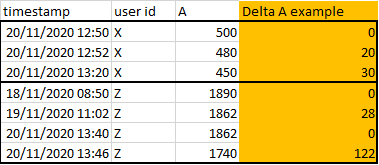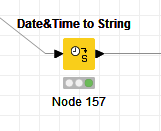# Calculate the difference between two rows of the same field sorting by timestamp

Hi!
I need to calculate the delta between two rows, for each row, taking into consideration the timestamp and for each user id.
I have these columns and I must calculate column Delta A example:For each user_id I have to:

• sort the timestamp (with sorter node)
• Then I have to calculate the difference for the column “A” between the timestamp 2 - timestamp 1, timestamp 3 - timestamp 2 etc…

How can I do that?
The first timestamp for each user id should be 0.

Thanks!

Hi,

Start with extracting date from the timestamp then use Group Loop start node (group is date and user ID). In the loop use Lag Column node and Math Formula node. After the Loop end node replace null values with 0 in the delta column (the first row in the group should be 0)

Thanks! I think there is something wrong in the node group loop start because If I set group as data and user id, I will obtain only one row in the entire file.
Otherwise If I set group every columns it works.

How can I do?
Thanks

Hi,

Have you first extracted the date from the timestamp.

Look at Select rows based on condition in precedents and followings rows … the problem is similar to yours

Yes, I have used the node Unix timestamp to date&time before the loop:Is it correct?

Thanks

Hi,
You have to delete the time from the date so the Groupby node can group by date (same day).

I use Extraxt Date&Time Fields nodeorbut you have to delete the time part

Ah ok but I need the time part because for each day and month I will have several records and I can distinguish them only from the time.

Otherwise, without the time, how can you identify which date is before or after one respect to another?

Thanks

You extract the date in new column the original will remain the same

Can you upload the excel file

Here the excel:
example.xlsx (18.9 KB)

I delete time from the date&time but it does not work: in the loop start node I always see one single row.

Thanks

Hi,

Here is a basic example on which you can work on

Hi! Thanks!
But I have two problems:

1. I Have “?” values in rta(-1). Why? I need all values
2. It seems it does not sort for time because if you see for example in column Delta A the value = -18, the time is at 10:04:41, and it is not after the previous one at 12:43:52… How can I sort for time?

Thanks again